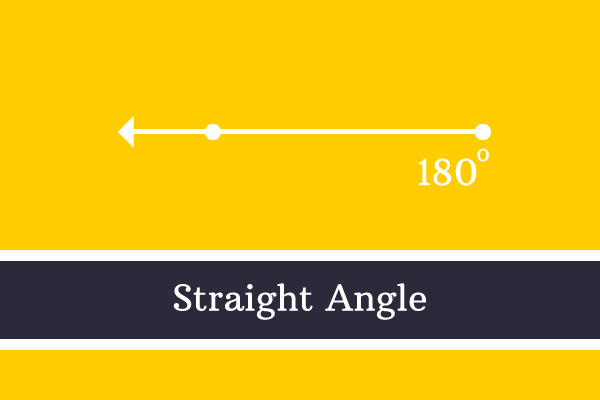# Straight angle## Definition

An angle of $180^°$ is called straight angle.

The meaning of straight angle is formed by the meanings of straight line and angle combinedly. In other words, if a straight line is formed in forming an angle then it is known as a straight line angle and simply called as straight angle.

### Representation

Straight line angle is denoted in three different systems according to angle measuring systems.

1. It is denoted by $180^°$ in Sexagesimal system
2. It is denoted by $\pi$ in Circular system
3. It is denoted by $200^g$ in Centesimal system

#### Case study

Straight line angles are possibly formed geometrically in three different cases.

1.
##### Straight angle by a lineAn angle of a straight line can be straight angle geometrically but it should follow two rules.

1. Line should be parallel to horizontal direction.
2. The starting point of line should be right or consider the right side point as starting point of the line.

$\overrightarrow{QR}$ is a ray on a plane. It is started from a point $Q$ and continued travelling infinitely through another point $R$ in left side direction of its starting point.

No other line can be considered as reference line to measure the angle of ray $\overrightarrow{QR}$. So, the horizontal direction is considered as reference line and measured the angle in anticlockwise direction.

The angle of ray $\overrightarrow{QR}$ with horizontal direction is $180^°$ and it is on a straight line. Hence, it is known as straight line angle and simply known as straight angle.

2.
##### Straight angle by rotationA straight angle is formed by a line geometrically and it is possible if a line is rotated to $180^°$ from its initial position.

For example

1. A ray $\overrightarrow{XY}$ is in a direction initially.
2. The ray is rotated to $180^°$ from its initial position and it appears as it is reflected oppositely from its initial position.
3. The ray $\overrightarrow{XY}$ is called $\overrightarrow{XZ}$ at its final position.

The ray made an angle of $180^°$ to reach its final position from its initial position.

It formed a straight line by combining the initial position and final position of the ray. Hence, it is called as straight angle and also straight line angle.

Note that the ray $\overrightarrow{XY}$ does not need to be parallel to horizontal reference line and it can make any angle. In this case, it is considered to measure an angle made by the ray from its initial position to final position and it is not the case about angle made by the horizontal reference line.

3.
##### Straight angle between linesTwo straight lines form a straight angle geometrically when the two straight lines have a common connecting point.

For example, $\overrightarrow{UV}$ and $\overrightarrow{UW}$ are two rays and they are formed by starting from point $U$ but locus of them in forming the angle formed a straight line.

The two rays form a straight line and the angle between them is $180^°$. Therefore, the angle is called as straight line angle but simply called as straight angle.

Note that the angle is measured by considering one ray as reference line to another and vice-versa to measure the angle between them and no need to consider the angle made by the straight line with horizontal reference line because it is the case about angle between two lines and not the case about angle made by the line.

Latest Math Topics
Jun 26, 2023
Jun 23, 2023

Latest Math Problems
Jul 01, 2023
Jun 25, 2023
###### Math Questions

The math problems with solutions to learn how to solve a problem.

Learn solutions

Practice now

###### Math Videos

The math videos tutorials with visual graphics to learn every concept.

Watch now

###### Subscribe us

Get the latest math updates from the Math Doubts by subscribing us.